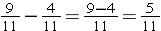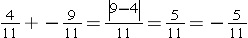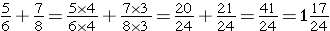In this lesson, you will extend your experience with addition and subtraction of fractions by adding and subtracting mixed numbers and by incorporating negative and positive fractions and mixed numbers into your expertise. Remember, the rules for addition and subtraction of fractions and mixed numbers are the same as integer rules.

## Rules

### Adding or Subtracting Fractions with Like Denominators

Add the numerators and keep the denominator. (Simplify)

1. Example2. Example3. ExampleInvestigate

Mr. Gordy walked ¼ mile to the library and then ¾ mile to the post office. What is the total distance he walked? Answer: one fourth plus three fourths equals four fourths. Four fourths equals one.

### Adding or Subtracting Fractions with Different Denominators

It is important to remember that fractions must have the same denominator before they can be added or subtracted. If they do not, then you will need to find a common denominator by finding the LCM. (Least common multiple) The LCM of two denominators is the lowest common denominator (LCD) of the fractions.

You can either find the LCM of the denominators or simply multiply the denominators.

Once fractions are converted, you can add and subtract the numerators and keep the new common denominator.

1. Example2. ExampleWhen you add or subtract mixed numbers, simply keep the whole numbers separate from the fractions. When you subtract, you may want to regroup.

(source)